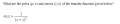# Bode Plots & High Pass, Low Pass, Band Stop and Pass Frequencies

#### libnitz

Joined Jan 29, 2016
17
Hi,

I just need some confirmation on 3 questions1. I was asked to calculate the poles and zeros. I believe

zeros: none
poles: 2 (or p1,2) = -1

2. I was also asked to calculate the slope of the magnitude response was w -> 0
Here is my sketched graphFrom my pic, it shows that as w -> 0, H(s) -> 0.

3. What type of filter response does this network exhibit.
I believe it will be a Low Pass because as

s->0, H(s) -> 1 and as s-> infinity, H(s) -> 0?

Does this reasoning make it a low pass filter, high pass, band stop or band pass?

Any help is appreciated

#### shteii01

Joined Feb 19, 2010
4,644
3.
Yes.
As w increases, s=jw will also increase. s is in the denominator so as s increases, the denominator also increases, so as s becomes bigger, $$\frac{1}{s^2}$$ will become smaller and smaller and smaller. So near 0 rad/s (or Hz) you will have some useful response, but as you move further away (toward infinity), the response will sharply drop.

•libnitz

#### libnitz

Joined Jan 29, 2016
17
3.
Yes.
As w increases, s=jw will also increase. s is in the denominator so as s increases, the denominator also increases, so as s becomes bigger, $$\frac{1}{s^2}$$ will become smaller and smaller and smaller. So near 0 rad/s (or Hz) you will have some useful response, but as you move further away (toward infinity), the response will sharply drop.
Thanks! I used the same reasoning as you wrote, however I was not too sure I got it right.

Could you also double check my calculations on this problem also?

MOD NOTE: You already have an active thread on this other problem.